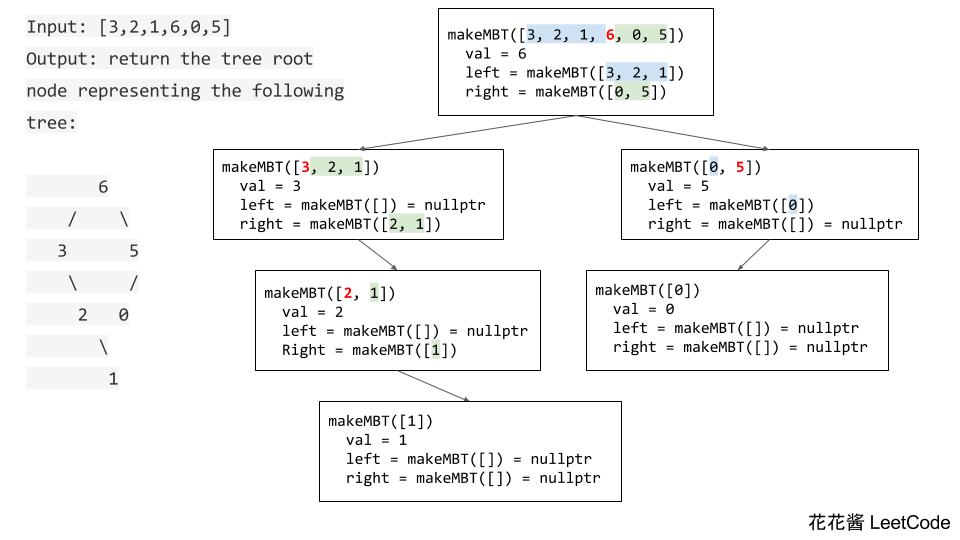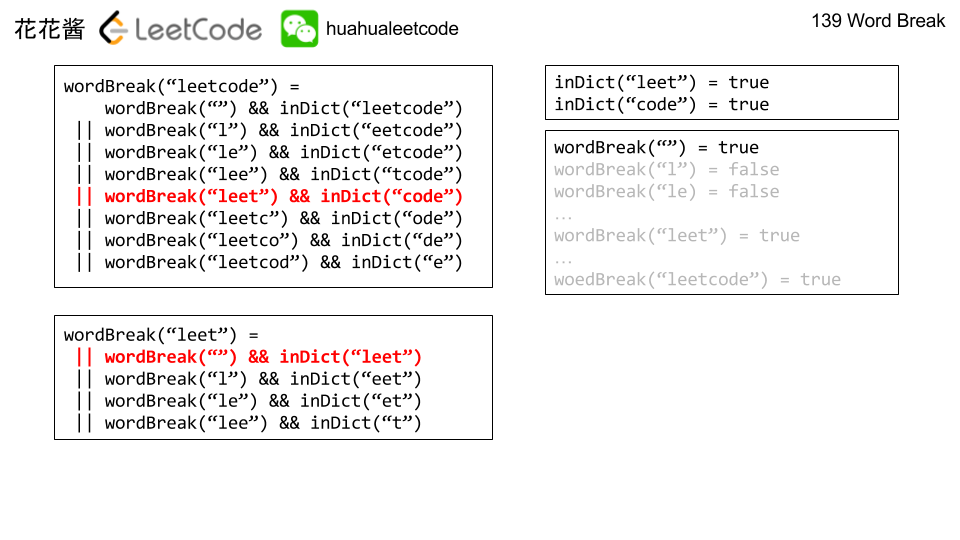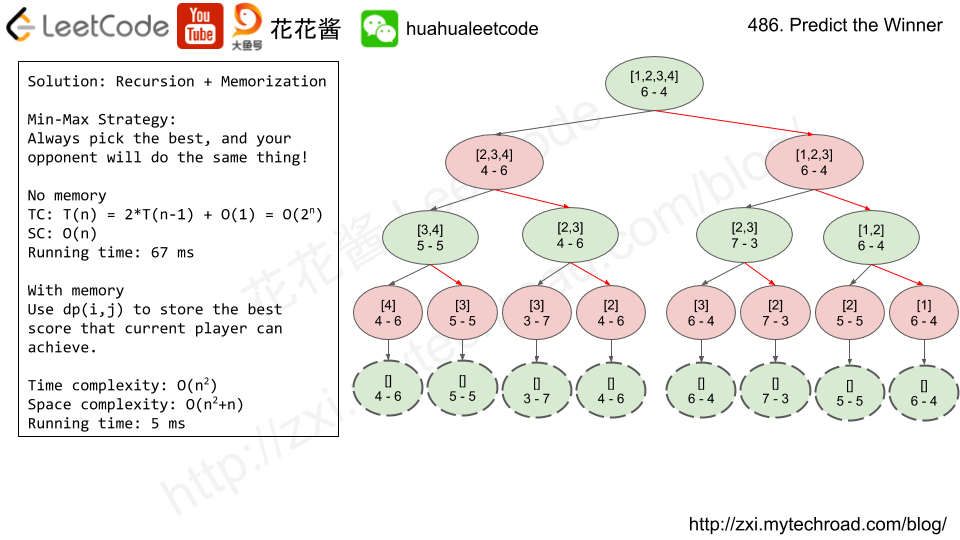Posts tagged as “medium”

Given an integer array with no duplicates. A maximum tree building on this array is defined as follow:

1. The root is the maximum number in the array.
2. The left subtree is the maximum tree constructed from left part subarray divided by the maximum number.
3. The right subtree is the maximum tree constructed from right part subarray divided by the maximum number.

Construct the maximum tree by the given array and output the root node of this tree.

Example 1:

Idea:

RecursionSolution:

With copy

Time complexity: O(nlogn) ~ O(n^2)

Space complexity: O(nlogn) ~ O(n^2)

running time 79ms

Without copy

Time complexity: O(nlogn) ~ O(n^2)

Space complexity: O(logn) ~ O(n)

running time 66ms

Merge two sorted linked lists and return it as a new list. The new list should be made by splicing together the nodes of the first two lists.

Solution 1: Iterative O(n)

Solution 2: Recursive O(n)

Problem

Given a non-empty string s and a dictionary wordDict containing a list of non-empty words, determine if s can be segmented into a space-separated sequence of one or more dictionary words. You may assume the dictionary does not contain duplicate words.

For example, given
s = "leetcode",
dict = ["leet", "code"].

Return true because "leetcode" can be segmented as "leet code".

UPDATE (2017/1/4):
The wordDict parameter had been changed to a list of strings (instead of a set of strings). Please reload the code definition to get the latest changes.

Idea:

DP

Time complexity O(n^2)

Space complexity O(n^2)Solutions:

C++

C++ V2 without using dict. Updated: 1/9/2018

Java

Python

Problem

Given an array of scores that are non-negative integers. Player 1 picks one of the numbers from either end of the array followed by the player 2 and then player 1 and so on. Each time a player picks a number, that number will not be available for the next player. This continues until all the scores have been chosen. The player with the maximum score wins.

Given an array of scores, predict whether player 1 is the winner. You can assume each player plays to maximize his score.

Example 1:
Input: [1, 5, 2]
Output: False
Explanation: Initially, player 1 can choose between 1 and 2.
If he chooses 2 (or 1), then player 2 can choose from 1 (or 2) and 5. If player 2 chooses 5, then player 1 will be left with 1 (or 2).
So, final score of player 1 is 1 + 2 = 3, and player 2 is 5.
Hence, player 1 will never be the winner and you need to return False.

Example 2:
Input: [1, 5, 233, 7]
Output: True
Explanation: Player 1 first chooses 1. Then player 2 have to choose between 5 and 7. No matter which number player 2 choose, player 1 can choose 233.
Finally, player 1 has more score (234) than player 2 (12), so you need to return True representing player1 can win.
Note:
1 <= length of the array <= 20.
Any scores in the given array are non-negative integers and will not exceed 10,000,000.
If the scores of both players are equal, then player 1 is still the winner.Solution 1: Recursion

Time complexity: O(2^n)

Space complexity: O(n)

Solution 2: Recursion + Memoization

Time complexity: O(n^2)

Space complexity: O(n)

DP version

Related Problem

Mission News Theme by Compete Themes.